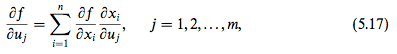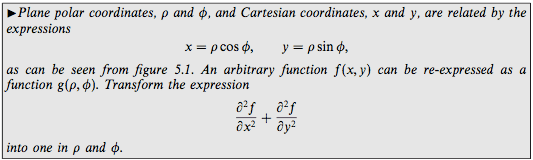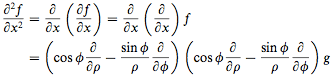# Proper notation in chain rule

• I
Is the chain rule below wrong?What I propose is as follows:

Given that ##x_i=x_i(u_1, u_2, ..., u_m)##. If we define the function ##g## such that ##g(u_1, u_2, ..., u_m)=f(x_1, x_2, ..., x_n)##, then

##\frac{\partial g}{\partial u_j}=\sum_{i=1}^n\frac{\partial f}{\partial x_i}\frac{\partial x_i}{\partial u_j}##.

This version of chain rule is what is being used, it seems, in the example below when the answer given replaces ##f## with ##g## in the last line.

A related question is as follows:

Consider the function ##V(r)=\frac{1}{3}\pi r^2h##, where ##h## is a constant. Suppose ##r## is a function of ##t## such that ##r(t)=at^2##, where ##a## is a constant.

What do we call the function after substituting ##r## with ##at^2##, which gives ##\frac{1}{3}\pi a^2t^4h##?

I guess we have to give it a different name: ##W(t)=\frac{1}{3}\pi a^2t^4h##, because ##V(t)## would give ##V(t)=\frac{1}{3}\pi t^2h##. Then ##\frac{\partial V(t)}{\partial t}=\frac{\partial V(r)}{\partial r}##. Am I right?

If we still call it ##V## as follows: ##V(t) = \frac 1 3 \pi a^2 t^4 h##, we will run into a problem.

Since ##V(r)=\frac{1}{3}\pi r^2h##, when ##r=2##, we would write ##V(2)=\frac{1}{3}\pi\,2^2\,h##. But if we write ##V(t)=\frac{1}{3}\pi a^2t^4h##, when ##t=2##, we have ##V(2)=\frac{1}{3}\pi a^2\,2^4\,h##. Then ##V(2)\neq V(2)##.Last edited:

About the first question I think the answer is that it is correct (the formula 5.17), but about
. Am I right?
The correct answer is the same, this one
##\frac{\partial V(t)}{\partial t}=\frac{\partial V(r)}{\partial r}\frac{\partial r}{\partial t}##
I did not check the last equation but I think it is not too difficult

Mark44
Mentor
Is the chain rule below wrong?
View attachment 102183

What I propose is as follows:

Given that ##x_i=x_i(u_1, u_2, ..., u_m)##. If we define the function ##g## such that ##g(u_1, u_2, ..., u_m)=f(x_1, x_2, ..., x_n)##, then

##\frac{\partial g}{\partial u_j}=\sum_{i=1}^n\frac{\partial f}{\partial x_i}\frac{\partial x_i}{\partial u_j}##.

This version of chain rule is what is being used, it seems, in the example below when the answer given replaces ##f## with ##g## in the last line.

A related question is as follows:

Consider the function ##V(r)=\frac{1}{3}\pi r^2h##, where ##h## is a constant. Suppose ##r## is a function of ##t## such that ##r(t)=at^2##, where ##a## is a constant.

What do we call the function after substituting ##r## with ##at^2##, which gives ##\frac{1}{3}\pi a^2t^4h##?
You still call it V, but now instead of V being a function of r, it's a function of t. You could refer to it as ##V(t) = \frac 1 3 \pi a^2 t^4 h##, or you could refer to it as ##V(r(t))##
Partials really don't have a place here. The first definition of V has it as a function of r alone (h is a constant, you said), so writing ##\frac{\partial V(r)}{\partial r}## is an overcomplication. ##\frac{d V(r)}{dr}## is appropriate.

Now, since r is a function of t alone, then you can refer to ##\frac{d V}{dt}##, and can calculate it using the chain rule for functions of a single variable.
Happiness said:
I guess we have to give it a different name: ##W(t)=\frac{1}{3}\pi a^2t^4h##, because ##V(t)## would give ##V(t)=\frac{1}{3}\pi t^2h##. Then ##\frac{\partial V(t)}{\partial t}=\frac{\partial V(r)}{\partial r}##. Am I right?

You still call it V, but now instead of V being a function of r, it's a function of t. You could refer to it as ##V(t) = \frac 1 3 \pi a^2 t^4 h##.

Since ##V(r)=\frac{1}{3}\pi r^2h##, when ##r=2##, we would write ##V(2)=\frac{1}{3}\pi\,2^2\,h##. But if we write ##V(t)=\frac{1}{3}\pi a^2t^4h##, when ##t=2##, we have ##V(2)=\frac{1}{3}\pi a^2\,2^4\,h##. Then ##V(2)\neq V(2)##.

Ssnow
Gold Member
Given that ##x_{i}=x_{i}(u_1,u_2,...,u_m)x_i=x_i(u_1, u_2, ..., u_m)##. If we define the function ##g## such that ##g(u_1,u_2,...,u_m)=f(x_1,x_2,...,x_n)##, then

I think it is understood also that you can express ##u_i=u_i(x_{1},\ldots,x_{n})##...

Mark44
Mentor
Since ##V(r)=\frac{1}{3}\pi r^2h##, when ##r=2##, we would write ##V(2)=\frac{1}{3}\pi\,2^2\,h##. But if we write ##V(t)=\frac{1}{3}\pi a^2t^4h##, when ##t=2##, we have ##V(2)=\frac{1}{3}\pi a^2\,2^4\,h##. Then ##V(2)\neq V(2)##.
No, that's not right. You're comparing apples and oranges. If r = 2, then V(2) = ##\frac{1}{3}\pi r^2h = \frac{1}{3}\pi 2^2h = \frac{4}{3}\pi h##. It's understood here that V(2) means that you evaluate things for r = 2.
V(r(2)) = V(2), but when r = 2 you're going to get a different value of V than for t = 2.

If r = 2, then ##t = \pm \sqrt{\frac 2 a}##, so V(r = 2) using the first formula is exactly equal to V(##t = \pm \sqrt{\frac 2 a}##) using the second formula.

This is basic function composition.

No, that's not right. You're comparing apples and oranges. If r = 2, then V(2) = ##\frac{1}{3}\pi r^2h = \frac{1}{3}\pi 2^2h = \frac{4}{3}\pi h##. It's understood here that V(2) means that you evaluate things for r = 2.
V(r(2)) = V(2), but when r = 2 you're going to get a different value of V than for t = 2.

If r = 2, then ##t = \pm \sqrt{\frac 2 a}##, so V(r = 2) using the first formula is exactly equal to V(##t = \pm \sqrt{\frac 2 a}##) using the second formula.

This is basic function composition.

So ##V(2)## could either mean ##V(r=2)## or ##V(t=2)##? And there is no universally accepted notation? It seems like this ambiguity can be avoided if we use good notations.

( ##V(r=2)## and ##V(t=2)## are themselves not good notations.)

Last edited:
Mark44
Mentor
So ##V(2)## could either mean ##V(r=2)## or ##V(t=2)##?
It should be clear from the context in which this is written.
Happiness said:
And there is no universally accepted notation? It seems like this ambiguity can be avoided if we use good notations.
Yes, of course.
If you write ##V(r) = \frac{1}{3}\pi r^2h##, and then also write ##V(t) = \frac{1}{3}\pi (at^2)^2h## (as you have done here), then writing V(2) is ambiguous. Does 2 represent a value of r or is it a value of t?

The first formulation of V above could be written as ##V(r(t)) = \frac{1}{3}\pi (r(t))^2h##, where ##r(t) = at^2##. That would clear up any ambiguity.

If you write ##V(r) = \frac{1}{3}\pi r^2h##, and then also write ##V(t) = \frac{1}{3}\pi (at^2)^2h## (as you have done here), then writing V(2) is ambiguous. Does 2 represent a value of r or is it a value of t?

If ##V## is a function that maps ##r## to ##\frac{1}{3}\pi r^2h##, then shouldn't it map ##t## to ##\frac{1}{3}\pi t^2h##? Then isn't ##V(t)=\frac{1}{3}\pi t^2h##?

This is the main reason why I believe we shouldn't write ##V(r(t))## as ##V(t)##. We should name it differently, for example, as ##W(t)##. Then ##V(2)## means ##r=2## and ##W(2)## means ##t=2##.

Mark44
Mentor
If ##V## is a function that maps ##r## to ##\frac{1}{3}\pi r^2h##, then shouldn't it map ##t## to ##\frac{1}{3}\pi t^2h##?
Yes, and V maps x to ##\frac{1}{3}\pi x^2h##, and it maps z to ##\frac{1}{3}\pi z^2h##, but so what?

What you seem to be forgetting is that there is a function composition going on, with V being a function of r, and r being a function of t. In an abuse of notation, we have V = V(r(t))
For a given value of t, find r(t), and then find V(r(t)). So if t = 2, r(2) = 4a, and V(r(2)) = ##\frac 4 3 \pi h##
Happiness said:
Then isn't ##V(t)=\frac{1}{3}\pi t^2h##?

This is the main reason why I believe we shouldn't write ##V(r(t))## as ##V(t)##. We should name it differently, for example, as ##W(t)##. Then ##V(2)## means ##r=2## and ##W(2)## means ##t=2##.
There's no need to give it a different name if you understand function composition.

Mark44
Mentor
Happiness said:
I guess we have to give it a different name: ##W(t)=\frac{1}{3}\pi a^2t^4h##, because ##V(t)## would give ##V(t)=\frac{1}{3}\pi t^2h##. Then ##\frac{\partial V(t)}{\partial t}=\frac{\partial V(r)}{\partial r}##.
I don't know why you're using partial derivatives. As this thread is about the chain rule, and your example is the composition of two functions of one variable, ordinary derivatives suffice. Given V in terms of r and r in terms of t, ##V'(t_0) = V'(r(t_0))*r'(t_0)##, or ##V'(t_0) = \left.\frac{dV}{dr}\right |_{r(t_0)} \left.\frac{dr}{dt} \right|_{t_0}##

Consider ##z## to be a function of ##y##, which is itself a function of ##x##. The chain rule may be written as ##\frac{dz}{dx}=\frac{dz}{dy}\frac{dy}{dx}##.

The ##z## on the LHS means ##z(y(x))##, which is equivalent to ##(z\circ y)(x)##, whereas the ##z## on the RHS means ##z(y)##. So strictly speaking, they are not the same, right? ##(z\circ y\neq z)##

Mark44
Mentor
Consider ##z## to be a function of ##y##, which is itself a function of ##x##. The chain rule may be written as ##\frac{dz}{dx}=\frac{dz}{dy}\frac{dy}{dx}##.
Sure. Here the context is that on the left side, we're talking about a map from x value to z values, and on the right side, the left derivative refers to a map from y values to z values. Certainly the two map formulas are different.
Happiness said:
The ##z## on the LHS means ##z(y(x))##, which is equivalent to ##(z\circ y)(x)##, whereas the ##z## on the RHS means ##z(y)##. So strictly speaking, they are not the same, right? ##(z\circ y\neq z)##

Some textbooks use a different function name. If x = g(t), and y = f(x), then they will define z = h(t) = f(g(t)). My point was that, as long as the context was understood, it wasn't necessary to introduce another function. If you have defined V in terms of r, then it's understood that V(2) means to evaluate V at r = 2. But if there is uncertainty whether you mean V as a function r or V as a function of t (with different formulas), then it's not clear what V(2) is supposed to mean.